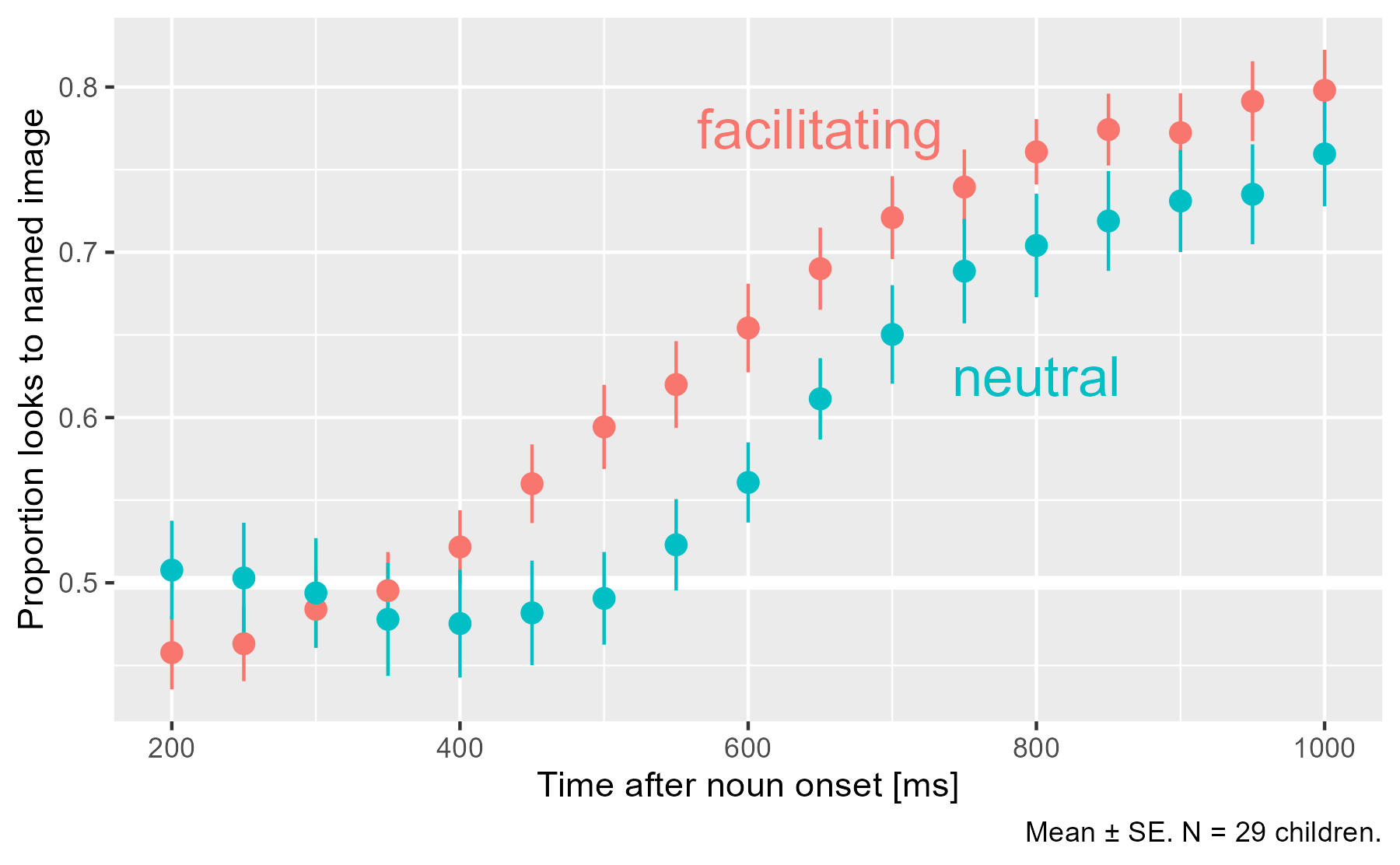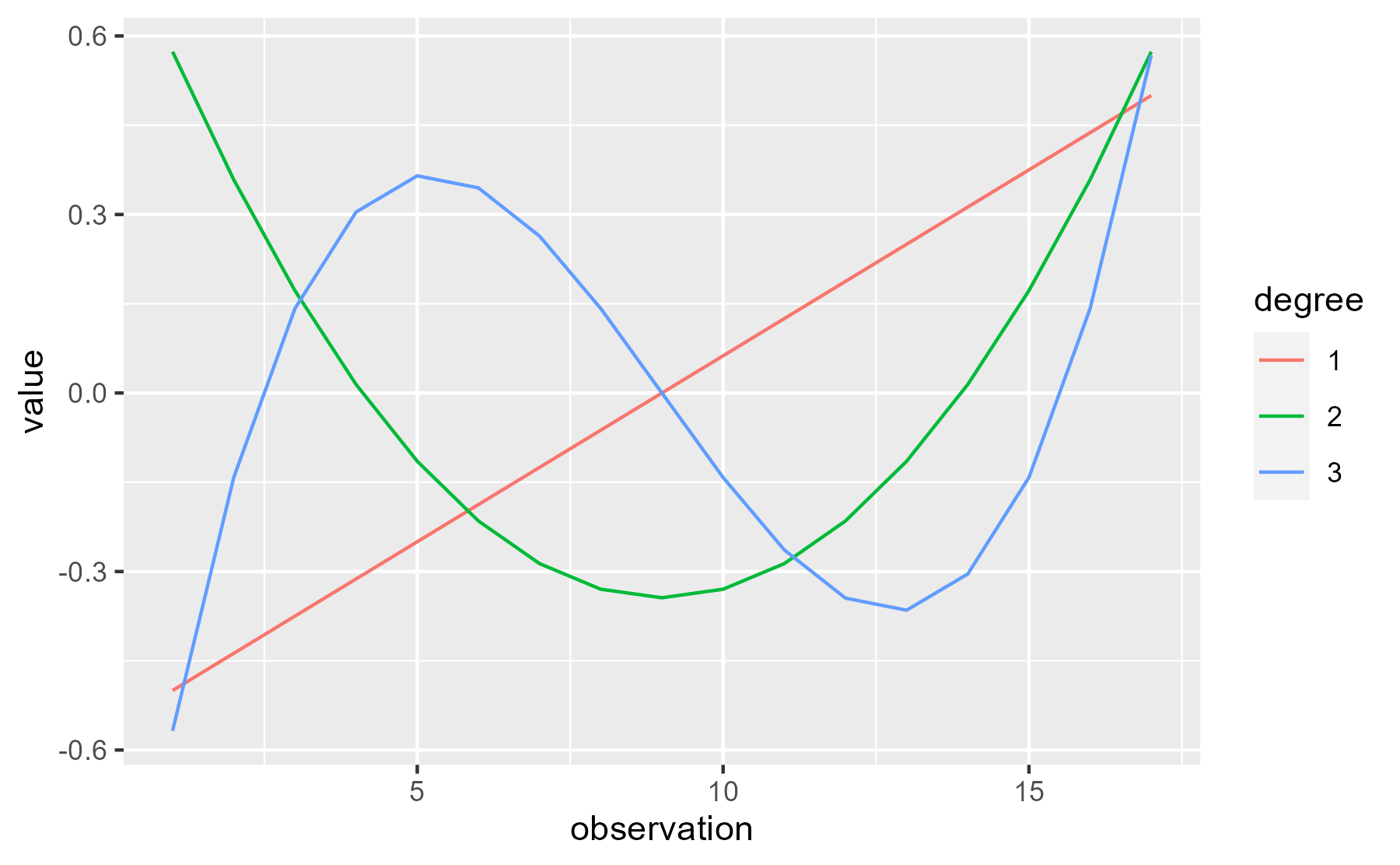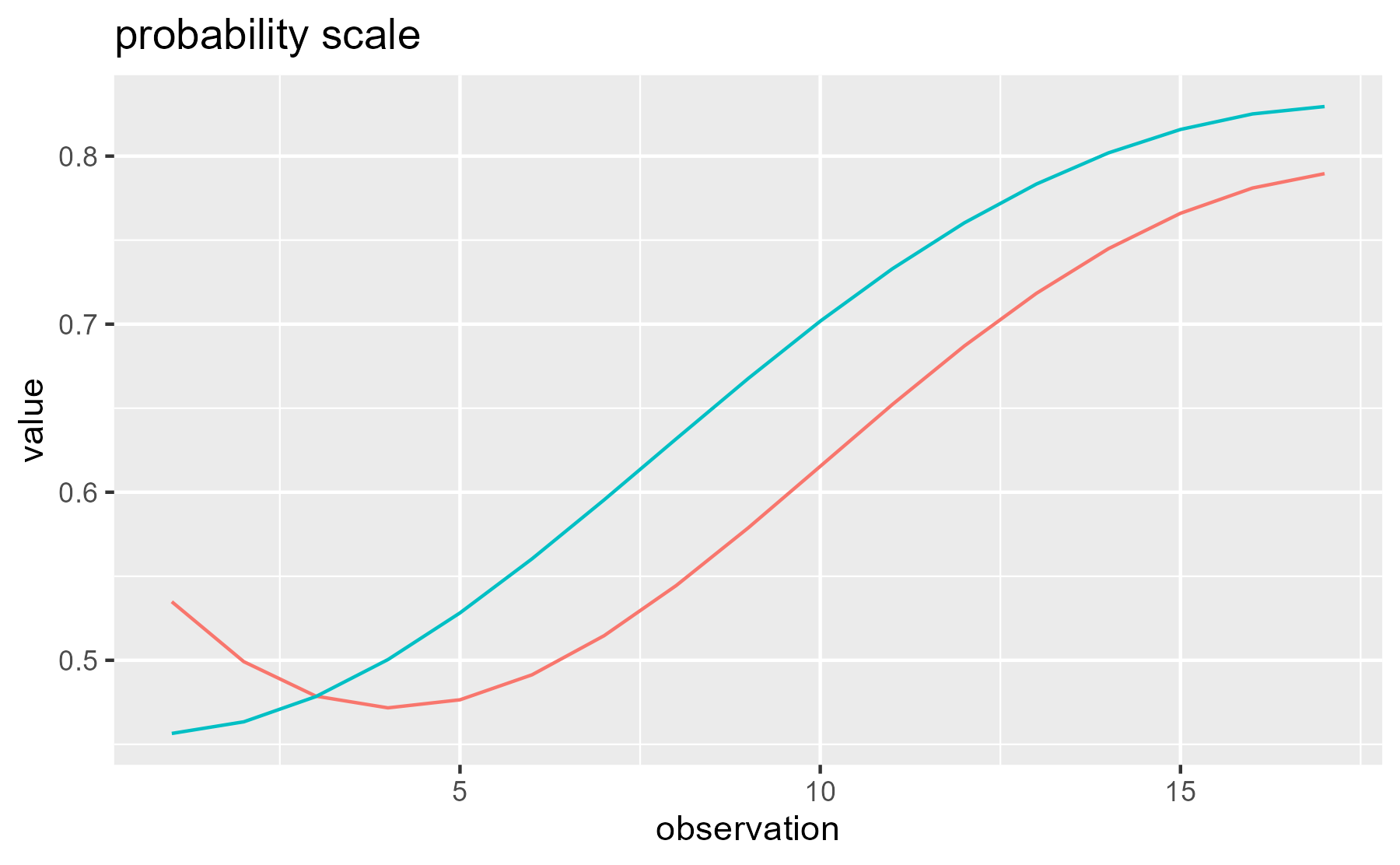# New package polypoly (helper functions for orthogonal polynomials)

May 29, 2017
By

Want to share your content on R-bloggers? click here if you have a blog, or here if you don't.

Last week, I released a new package called polypoly to CRAN. It wraps up
some common tasks for dealing with orthogonal polynomials into a single package.
The README shows off the main
functionality, as well as the neat “logo” I made for the package.
In this post, I use the package on some word recognition data.

## Demo: Growth curve analysis

I primarily use orthogonal polynomials to model data from eyetracking
experiments where growth curves describe how the probability of looking at a
image changes as the image is named. The analysis technique, including
orthogonal polynomials and mixed effects models of eyetracking data, are
described in Mirman’s 2014 book.

In our 2015 paper, toddlers saw
two images on a computer screen. The objects in the images started with
different consonants: for example, duck and ball. The toddlers heard
sentences like “find the ball”, and we measured how their gaze location onscreen
changed in response to speech. This setup is a pretty standard procedure for
studying spoken word recognition.

We manipulated the vowel in the word the. In the facilitating condition, the
vowel has acoustic information (via anticipatory coarticulation) which would
allow an adult listener to predict the upcoming consonant. In the neutral
condition, the vowel provides no cues about the upcoming consonant. The
scientific question is whether these kiddos can take advantage of these acoustic
cues during word recognition.

Here’s how the data look, both in R and in a plot.

``````library(ggplot2)
library(dplyr)

# The data
d
#> # A tibble: 986 x 6
#>     Subj    Condition  Time ToDistractor ToTarget Proportion
#>
#>  1     1 facilitating   200            9        9  0.5000000
#>  2     1 facilitating   250            9       10  0.5263158
#>  3     1 facilitating   300            6       12  0.6666667
#>  4     1 facilitating   350            6       12  0.6666667
#>  5     1 facilitating   400            6       12  0.6666667
#>  6     1 facilitating   450            6       12  0.6666667
#>  7     1 facilitating   500            6       12  0.6666667
#>  8     1 facilitating   550            6       12  0.6666667
#>  9     1 facilitating   600            4       12  0.7500000
#> 10     1 facilitating   650            3       15  0.8333333
#> # ... with 976 more rows

# Helper dataframe of where to put condition labels on the next plot
df_labs <- data_frame(
Time = c(650, 800),
Proportion = c(.775, .625),
Condition = c("facilitating", "neutral"))

p <- ggplot(d) +
aes(x = Time, y = Proportion, color = Condition) +
geom_hline(yintercept = .5, size = 2, color = "white") +
stat_summary(fun.data = mean_se) +
geom_text(aes(label = Condition), data = df_labs, size = 6) +
labs(x = "Time after noun onset [ms]",
y = "Proportion looks to named image",
caption = "Mean ± SE. N = 29 children.") +
guides(color = "none")
p
``````Early on, children look equal amounts to both images on average (.5), and the
proportion of looks to the named image increase as the word unfolds. In the
facilitating condition, that rise happens earlier.

We fit a mixed-effects logistic regression model to estimate how the probability
of looking to the named image changes over time, across conditions, and within
children. We use cubic orthogonal polynomials to represent Time. For each time
point, we have three predictors available to us: Time1,
Time2, and Time3. (Plus, there’s a constant “intercept”
term.) Our model’s growth curve will be a weighted combination of these polynomial
curves. The code below shows off about half the functionality of the package
:bowtie::

``````poly(unique(d\$Time), 3) %>%
# Force Time^1 term to range from -.5 to .5. Rescale others accordingly.
polypoly::poly_rescale(scale_width = 1) %>%
polypoly::poly_plot()
``````I think people sometimes describe the contributions of these curves to the
overall growth curve as trends: “A negative linear trend”, “a significant
quadratic trend”, etc. I like that word because it makes the terminology a
little less intimidating.

### Quick aside: Why orthogonal polynomials?

Why do we use orthogonal polynomial terms? First, note that simple polynomials
x, x2 and x3 are correlated. Orthogonal ones are not
correlated. (Hence, the name.)

``````# Simple
poly(1:10, 3, raw = TRUE) %>%
cor() %>%
round(2)
#>      1    2    3
#> 1 1.00 0.97 0.93
#> 2 0.97 1.00 0.99
#> 3 0.93 0.99 1.00

# Orthogonal
poly(1:10, 3, raw = FALSE) %>%
cor() %>%
round(2)
#>   1 2 3
#> 1 1 0 0
#> 2 0 1 0
#> 3 0 0 1
``````

Adding new correlated predictors to a model is a problem. The parameter
estimates will change as different predictors are added. Here we simulate some
fake data, and fit three models with 1-, 2- and 3-degree raw polynomials.

``````x <- 1:10
y <- x +
rnorm(1, mean = 100) * (x) +
rnorm(1, mean = 0, sd = .01) * (x) ^ 2 +
rnorm(1, mean = -1) * (x) ^ 3 +
rnorm(10)

models <- list(
m1 = lm(y ~ x),
m2 = lm(y ~ x + I(x^2)),
m3 = lm(y ~ x + I(x^2) + I(x^3))
)
``````

As expected, the estimates for the effects change from model to model:

``````models %>%
lapply(broom::tidy) %>%
bind_rows(.id = "model") %>%
select(model:estimate) %>%
mutate(estimate = round(estimate, 2))
#>   model        term estimate
#> 1    m1 (Intercept)    75.69
#> 2    m1           x    72.91
#> 3    m2 (Intercept)   -23.91
#> 4    m2           x   122.72
#> 5    m2      I(x^2)    -4.53
#> 6    m3 (Intercept)     1.15
#> 7    m3           x   100.48
#> 8    m3      I(x^2)     0.29
#> 9    m3      I(x^3)    -0.29
``````

But with orthogonal polynomials, the parameter estimates don’t change from model
to model.

``````models2 <- list(
m1 = lm(y ~ poly(x, 1)),
m2 = lm(y ~ poly(x, 2)),
m3 = lm(y ~ poly(x, 3))
)

models2 %>%
lapply(broom::tidy) %>%
bind_rows(.id = "model") %>%
select(model:estimate) %>%
mutate(estimate = round(estimate, 2))
#>   model        term estimate
#> 1    m1 (Intercept)   476.72
#> 2    m1  poly(x, 1)   662.27
#> 3    m2 (Intercept)   476.72
#> 4    m2 poly(x, 2)1   662.27
#> 5    m2 poly(x, 2)2  -104.03
#> 6    m3 (Intercept)   476.72
#> 7    m3 poly(x, 3)1   662.27
#> 8    m3 poly(x, 3)2  -104.03
#> 9    m3 poly(x, 3)3   -16.24
``````

That’s probably the simplest reason why orthogonal polynomials are preferred. (I
can’t remember any others right now.)

### Back to the data

Before fitting the model, I use `poly_add_columns()` to add polynomial terms as
columns to the dataframe. (For speed here, I use a simplified random effects
structure, estimating growth curve parameters for each Child x Condition
combination.)

``````library(lme4)

d <- d %>%
polypoly::poly_add_columns(Time, degree = 3, prefix = "ot", scale_width = 1) %>%
# Change the reference level
mutate(Condition = factor(Condition, c("neutral", "facilitating")))

m <- glmer(
cbind(ToTarget, ToDistractor) ~
(ot1 + ot2 + ot3) * Condition +
(ot1 + ot2 + ot3 | Subj:Condition),
family = binomial,
data = d)
``````

We can confirm that the model captures the overall shape of the growth curves.

``````# The lines here are not quite the overall average, but the averages of 29
# individual fits (for each participant). That's why the caption is a little
# weird.
p +
stat_summary(aes(y = fitted(m)), fun.y = mean, geom = "line") +
labs(caption = "Line: Average of model-fitted values. Points: Mean ± SE.")
``````We can inspect the model summary as well.

``````arm::display(m)
#> glmer(formula = cbind(ToTarget, ToDistractor) ~ (ot1 + ot2 +
#>     ot3) * Condition + (ot1 + ot2 + ot3 | Subj:Condition), data = d,
#>     family = binomial)
#>                           coef.est coef.se
#> (Intercept)                0.47     0.10
#> ot1                        1.57     0.28
#> ot2                        0.45     0.11
#> ot3                       -0.34     0.09
#> Conditionfacilitating      0.23     0.14
#> ot1:Conditionfacilitating  0.45     0.39
#> ot2:Conditionfacilitating -0.44     0.16
#> ot3:Conditionfacilitating  0.11     0.13
#>
#> Error terms:
#>  Groups         Name        Std.Dev. Corr
#>  Subj:Condition (Intercept) 0.53
#>                 ot1         1.46      0.23
#>                 ot2         0.52     -0.05  0.31
#>                 ot3         0.39     -0.08 -0.64  0.09
#>  Residual                   1.00
#> ---
#> number of obs: 986, groups: Subj:Condition, 58
#> AIC = 4788.2, DIC = -3961.1
#> deviance = 395.6
``````

The model summary indicates a significant Condition x Time2
interaction, but really, only the intercept and Time1 can ever be
interpreted directly. To understand the model fit, we visualize how each of the
polynomial terms are weighted.

Here we create a matrix of the polynomial terms plus a column of ones for the
intercept.

``````time_mat <- poly(sort(unique(d\$Time)), 3) %>%
polypoly::poly_rescale(1) %>%
cbind(constant = 1, .)
round(time_mat, 2)
#>       constant     1     2     3
#>  [1,]        1 -0.50  0.57 -0.57
#>  [2,]        1 -0.44  0.36 -0.14
#>  [3,]        1 -0.37  0.17  0.14
#>  [4,]        1 -0.31  0.01  0.30
#>  [5,]        1 -0.25 -0.11  0.36
#>  [6,]        1 -0.19 -0.22  0.34
#>  [7,]        1 -0.12 -0.29  0.26
#>  [8,]        1 -0.06 -0.33  0.14
#>  [9,]        1  0.00 -0.34  0.00
#> [10,]        1  0.06 -0.33 -0.14
#> [11,]        1  0.12 -0.29 -0.26
#> [12,]        1  0.19 -0.22 -0.34
#> [13,]        1  0.25 -0.11 -0.36
#> [14,]        1  0.31  0.01 -0.30
#> [15,]        1  0.37  0.17 -0.14
#> [16,]        1  0.44  0.36  0.14
#> [17,]        1  0.50  0.57  0.57
``````

To compute the weighted values, we multiply by a diagonal matrix of the
coefficients.

``````neut_coefs <- fixef(m)[1:4]
faci_coefs <- neut_coefs + fixef(m)[5:8]
faci_coefs
#>  (Intercept)          ot1          ot2          ot3
#>  0.699926322  2.014186150  0.006646146 -0.226658408

set_colnames <- `colnames<-`

m_neut <- time_mat %*% diag(neut_coefs) %>%
set_colnames(c("constant", "ot1", "ot2", "ot3"))

m_faci <- time_mat %*% diag(faci_coefs) %>%
set_colnames(c("constant", "ot1", "ot2", "ot3"))

# Convince ourselves with an example
round(m_faci, 2)
#>       constant   ot1 ot2   ot3
#>  [1,]      0.7 -1.01   0  0.13
#>  [2,]      0.7 -0.88   0  0.03
#>  [3,]      0.7 -0.76   0 -0.03
#>  [4,]      0.7 -0.63   0 -0.07
#>  [5,]      0.7 -0.50   0 -0.08
#>  [6,]      0.7 -0.38   0 -0.08
#>  [7,]      0.7 -0.25   0 -0.06
#>  [8,]      0.7 -0.13   0 -0.03
#>  [9,]      0.7  0.00   0  0.00
#> [10,]      0.7  0.13   0  0.03
#> [11,]      0.7  0.25   0  0.06
#> [12,]      0.7  0.38   0  0.08
#> [13,]      0.7  0.50   0  0.08
#> [14,]      0.7  0.63   0  0.07
#> [15,]      0.7  0.76   0  0.03
#> [16,]      0.7  0.88   0 -0.03
#> [17,]      0.7  1.01   0 -0.13
``````

Then, we can use the `poly_melt()` function to get a dataframe from each
weighted matrix and then plot each of the effects.

``````df_neut <- m_neut %>%
polypoly::poly_melt() %>%

df_faci <- m_faci %>%
polypoly::poly_melt() %>%

df_both <- bind_rows(df_faci, df_neut) %>%
mutate(Condition = factor(Condition, c("neutral", "facilitating")))

ggplot(df_both) +
aes(x = observation, y = value, color = Condition) +
geom_line() +
facet_wrap("degree")
``````Visually, the quadratic effect on the neutral curve pulls down the values during
the center (when the curves are most different) and pushes the values in the
tails upwards (when the curves are closest). Although only the quadratic effect
is nominally significant, the constant and linear terms suggest other smaller
effects but they are too noisy to pin down.

It’s worth noting that the predictors and weights discussed above are on the
log-odds/logit scale used inside of the model, instead of the proportion scale
used in the plots of the data and model fits. Basically, these weighted values
are summed together and then squeezed into the range [0, 1] with a nonlinear
transformation. For these data, the two scales produce similar looking growth
curves, but you can notice that the right end of the curves are pinched slightly
closer together in the probability-scale plot:

``````ggplot(df_both) +
aes(x = observation, y = value, color = Condition) +
stat_summary(fun.y = sum, geom = "line") +
ggtitle("logit scale") +
guides(color = "none")

ggplot(df_both) +
aes(x = observation, y = value, color = Condition) +
stat_summary(fun.y = function(xs) plogis(sum(xs)), geom = "line")  +
ggtitle("probability scale") +
guides(color = "none")
``````R-bloggers.com offers daily e-mail updates about R news and tutorials about learning R and many other topics. Click here if you're looking to post or find an R/data-science job.
Want to share your content on R-bloggers? click here if you have a blog, or here if you don't.